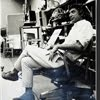## Eugene Ray SDSU 1970'sEugene Ray 1970's. photo credit: Tom Davis

## Friday, December 7, 2012

### ANCIENT ARCHITECTURE temple provides link w/ eternal cosmos ^^^^^^^^^^^^^^^^^^^^^ SPHINX-EXTRATERRESTRIAL link may explain many u/a questions ^^^^^^^^^^^^^^^^^^^^^ THE SPHINX MYSTERY forgotten origins of the sanctuary ANUBIS (robert temple w/ olivia temple) ^^^^^^^^^^^^^^^^^^^^^ author of "SYRIUS MYSTERY" w/ a new INTENSE RESEARCH book Inbox x+GRATITUDE+
>to mary lynn hyde, tulane alumni<
>official and good friend ref to<
+OSCAR NIEMEYER+
>master brazilian architect and<
+DESIGNER OF BRAZILIA+
(>locus of my last international<
>color slide/oeuvre lecture<)
^^^^^^^^^^^^^^
+DIED IN RIO de JANEIRO+
>december fifth<
+AT AGE 104 YEARS+!
^^^^^^^^^
>oscar niemeyer was a fabulous<
>designer w/ a passion for<
+CURVED STRUCTURE+
^^^^^^^^^^^
>which may explain why i was so<
>pleased when he wrote to one<
>of my grad students in the<
+1990s (KIND NOTE)+
^^^^^^^^^
>i dedicate tonight's message<
>to oscar niemeyer, architect<
^^^^^^^^^^^^^^^

+THE SPHINX AS A TEMPLE+
>ancient et/egypt<
^^^^^^^^^^

+3500 BC+
>american/british research professor<
+ROBERT TEMPLE+
>wrote  in his early famous book<
+"THE SYRIUS MYSTERY"+
>is when the african dogon people<
>(descended from egyptian)<
^^^^^^^^^^^^^^
+BELIEVE ANCIENT ALIENS CAME+
+TO PLANET EARTH IN EGYPT+
+SYRIA & BABYLONIA+
^^^^^^^^^^
>this may be profound knowledge<
>to support the research of+
+CHRISTOPHER DUNN+
+WUN CHOK BONG+
>and many others regarding<
>ancient alien interface in<
+ANCIENT EGYPT+
^^^^^^^^

>now robert temple has a new book<
>written w/ the aid of wife olivia<
^^^^^^^^^^^^^^^^^
+"THE SPHINX MYSTERY"+
(>"the forgotten origins of the<
+SANCTUARY OF ANUBIS"+)
^^^^^^^^^^^^^
+ESOTERIC LIBRARY+
>is strong revelation in the profound<
>architectural structure found in<
+ANCIENT EGYPT+
^^^^^^^^^

+TONIGHT'S PHOTOS+
>five photos reveal robert temples<
>conclusion-the sphinx is the<
>on the body of a<
+D O G+ !
^^^
>not a lion as commonly thought>
+ANUBIS JACKEL+
>could this explain the tribe<
+DOGON+
>(no pun intended here)<
^^^^^^^^^^^^

+O V E R V I E W+
>how could the dogon tribe w/o<
+SYRIUS STARS+
>and extraterrestrial history<
^^^^^^^^^^^^^^^^
+T R I B U T E+
>eric von daniken, father of the<
+ANCIENT ALIENS+
>television program series<
^^^^^^^^^^^^^^

+A F T E R+N O T E+
>as one who has collected cia (ex?)<
>agent friends in large number<
>i was shocked to discover<
+ROBERT TEMPLE+
>and<
+ROD SERLING+
>both were studied by the cia<
>apparently for their<
^^^^^^^^^^
+ANCIENT ALIEN RESEARCH+
^^^^^^^^^^^^^^^
(>note : the freedom of information<
>act does not guarantee release<
>of all secret cia documents<)
^^^^^^^^^^^^^^

+FASCINATING REALITY+
>governments are very interested<
(+#1 TOP SECRET+)
>in extraterrestrial research<
^^^^^^^^^^^^^^^
+GALVENISES UFO RESEARCH+
+AND MY PERSONAL LIFE+

+INVOLVMENT+
^^^^^^
http://www.TheTruthBehindTheScenes.org/2012/12/07/travel-channel-to-explore-ufo-debris-from-the-russian-roswell-incident/
gene ray, lajolla, california

>eugene ray mfa, architect<
>professor emeritus, sdsu,
^^^^^^^^^^^^^